## Gauss-Bonnet Formula

The Gauss-Bonnet formula has several formulations. The simplest one expresses the total Gaussian Curvature of an embedded triangle in terms of the total Geodesic Curvature of the boundary and the Jump Angles at the corners.

More specifically, if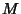is any 2-D Riemannian Manifold (like a surface in 3-space) and if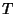is an embedded triangle, then the Gauss-Bonnet formula states that the integral over the whole triangle of the Gaussian Curvature with respect to Area is given by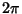minus the sum of the Jump Angles minus the integral of the Geodesic Curvature over the whole of the boundary of the triangle (with respect to Arc Length),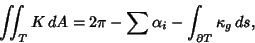(1)

where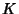is the Gaussian Curvature,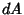is the Area measure, the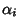s are the Jump Angles of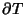, and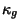is the Geodesic Curvature of, with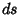the Arc Length measure.

The next most common formulation of the Gauss-Bonnet formula is that for any compact, boundaryless 2-D Riemannian Manifold, the integral of the Gaussian Curvature over the entire Manifold with respect to Area istimes the Euler Characteristic of the Manifold,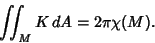(2)

This is somewhat surprising because the total Gaussian Curvature is differential-geometric in character, but the Euler Characteristic is topological in character and does not depend on differential geometry at all. So if you distort the surface and change the curvature at any location, regardless of how you do it, the same total curvature is maintained.

Another way of looking at the Gauss-Bonnet theorem for surfaces in 3-space is that the Gauss Map of the surface has Degree given by half the Euler Characteristic of the surface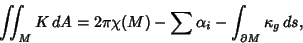(3)

which works only for Orientable Surfaces. This makes the Gauss-Bonnet theorem a simple consequence of the Poincare-Hopf Index Theorem, which is a nice way of looking at things if you're a topologist, but not so nice for a differential geometer. This proof can be found in Guillemin and Pollack (1974). Millman and Parker (1977) give a standard differential-geometric proof of the Gauss-Bonnet theorem, and Singer and Thorpe (1996) give a Gauss's Theorema Egregium-inspired proof which is entirely intrinsic, without any reference to the ambient Euclidean Space.

A general Gauss-Bonnet formula that takes into account both formulas can also be given. For any compact 2-D Riemannian Manifold with corners, the integral of the Gaussian Curvature over the 2-Manifold with respect to Area istimes the Euler Characteristic of the Manifold minus the sum of the Jump Angles and the total Geodesic Curvature of the boundary.

References

Chavel, I. Riemannian Geometry: A Modern Introduction. New York: Cambridge University Press, 1994.

Guillemin, V. and Pollack, A. Differential Topology. Englewood Cliffs, NJ: Prentice-Hall, 1974.

Millman, R. S. and Parker, G. D. Elements of Differential Geometry. Prentice-Hall, 1977.

Reckziegel, H. In Mathematical Models from the Collections of Universities and Museums (Ed. G. Fischer). Braunschweig, Germany: Vieweg, p. 31, 1986.

Singer, I. M. and Thorpe, J. A. Lecture Notes on Elementary Topology and Geometry. New York: Springer-Verlag, 1996.